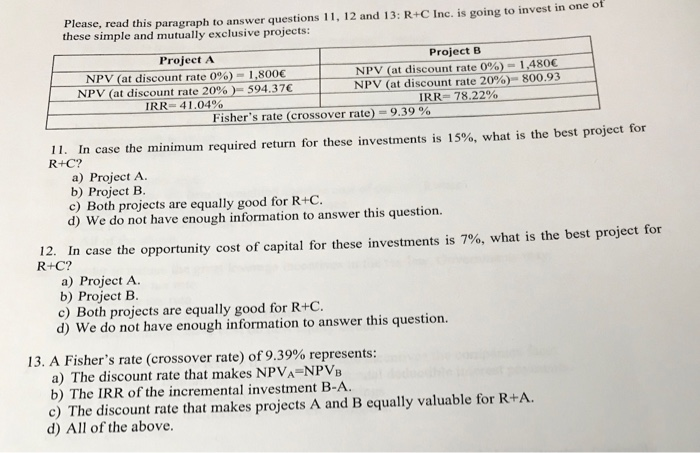# Sensitivity Analysis and Scenario Analysis Assignment10. Sensitivity analysis and Scenario analysis are methods that:

a) Deal with risk that comes from the project cash flows.

b) Deal with risk that comes from the project opportunity cost of capital.

c) Plot the NPV as a function of the discount rate

d) Study the minimum number of units the company has to sell to get positive NPV.

Please, read this paragraph these simple and mutually exclusive projects: to answer questions 11, 12 and 13: R+C Inc, is going to invest in one of Project B Project A NPV (at discount rate 0%)- NPV (at discount rate 20% ) – 594.37 IRR- 41.04 % 1,480 NPV (at discount rate 0%) NPV (at discount rate 20% ) – 800.93 IRR- 78.22% 1,800 Fisher’s rate (crossover rate)- 9.39 %

11. In case the minimum required return for these investments is 15% , what is the best project for R+C?

a) Project A.

b) Project B

c) Both projects are equally good for R+C

d) We do not have enough information to answer this question.

12. In case the opportunity cost of capital for these investments is 7%, what is the best project for R+C?

a) Project A

b) Project B

c) Both projects are equally good for R+C.

d) We do not have enough information to answer this question. 13. A Fisher’s rate (crossover rate) of 9.39% represents:

a) The discount rate that makes NPVA=NPVB

b) The IRR of the incremental investment B-A

c) The discount rate that makes projects A and B equally valuable for R+A

d) All of the above. Get Finance homework help today